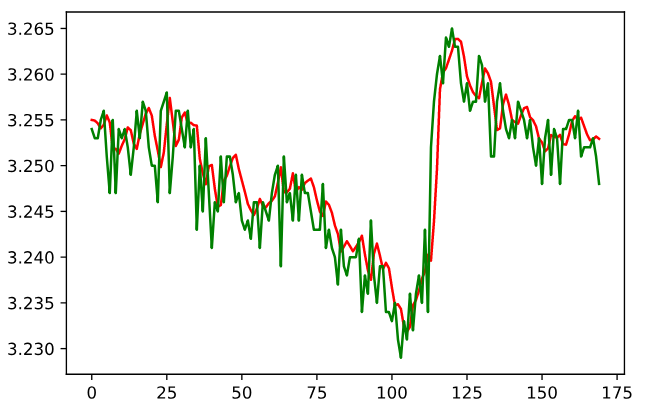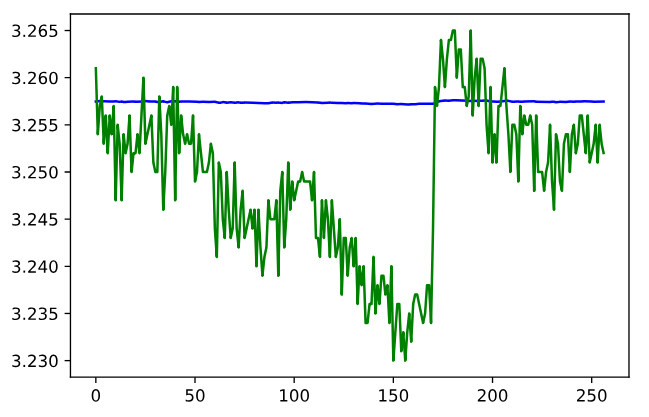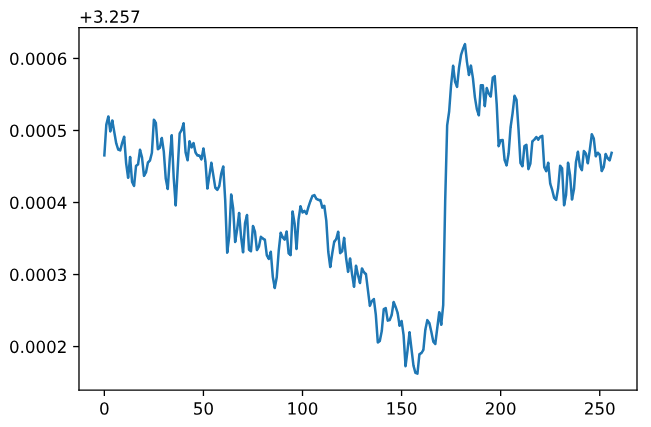# 【Time Series 】 The prediction result of LSTM is approximately straight line

Hi,

Recently, I was working on a time series prediction project, using the RNN and LSTM modules of Pytorch.

I have a problem. When I use RNN, the prediction results are acceptable. But when I use LSTM, I get very poor results. 【PS:I use the same data structure, parameter structure, on RNN and LSTM.】

I try to change the amount of data per training, the number of hidden neurons and the number of layers in LSTM, but the predicted results can not fit the real data well.

Here are the prediction results I got：The above graph shows the RNN prediction results. The `green line` represents the` real data`, and the `red line` represents the` prediction result`. I am quite satisfied with the result
NextThe above graph shows the LSTM prediction results.The `green line` represents the` real data`, and the `blue line` represents the` prediction result`. As you can see, the predicted result is almost a straight line.
When I zoom in on the prediction results， I found that the trend was like this.【I don’t know why this is happening? Is there any solution that can help me solve this problem.】
——
Here are the main code snippets for LSTM

``````#every time, use the data of three time points  to predict the data of the next one time
use_data=torch.Size([1790, 1, 3])
back_data=torch.Size([1790, 1, 1])

BATCH_SIZE=1
LR = 0.0003
EPOCHS = 10

train_set=data.TensorDataset(use_data,back_data)

class lstm(nn.Module):
def __init__(self):
super(lstm, self).__init__()
self.lstm = nn.LSTM(3,3)
self.linear = nn.Linear(3, 1)

def forward(self, x,h):
y1, h = self.lstm(x,h)
y3 = self.linear(y1)
return y3,h

NET = lstm()
loss_func = nn.MSELoss()

h_state = torch.randn(1,1,3)
c_state=torch.randn(1,1,3)
hx=(h_state,c_state)

**lstm(
(lstm): LSTM(3, 3)
(linear): Linear(in_features=3, out_features=1, bias=True)
)

total_loss=[]
wc_loss_plt=[]

NET.train()
for step in range(EPOCHS):
wc_loss=[]
pre=[]
for i, (batch_x, batch_y) in enumerate(loader):
out, hx = NET(batch_x,hx)

hx1=hx.detach()
hx2=hx.detach()
hx=(hx1,hx2)

loss = loss_func(out, batch_y)
pre.append(out)    ### prediction result
wc_loss.append(loss.data)

loss.backward()
optimizer.step()

total_loss.append(sum(wc_loss))
``````

You might need to continue the training. Since the predictions seem to already take the shape of the target the scaling might still need to be adjusted.
How are the training and validation loss behaving? Are both still decreasing or are you seeing a plateau?

1 Like